# Repeatability, Uncertainty and Accuracy of Flow Meters

While talking about flow measurement, a variety of terms are critical to take into consideration. They include Repeatability, Uncertainty, Accuracy and Turndown.

## Repeatability

Repeatability is the ability of a flow meter to show the same value for an identical flow rate on more than one occasion.

Having optimal repeatability is critical. But, this does not decrease the importance of accuracy under any conditions.

## Uncertainty

‘Uncertainty’ nowadays is becoming a more popular term than accuracy because accuracy cannot be established, as the true value can never be precisely known.

However ‘uncertainty’ can be calculated and an ISO standard exists regarding this matter (EN ISO/IEC 17025). It is crucial to know that it's a statistical idea and not a guarantee.

For example, it could be proven that with a high population of flow meters, 95% would be at least as appropriate as the uncertainty measured. Most might be much better, but a few, 5% may be worse.

## Accuracy

It determines how precise flow measurement devices are to the actual value or flow rate and is used to determine the suitability of the flow meter for a specific application.

Flow meter accuracy statements are expressed as a percent of either:

• Flow rate (%R)
• Full scale (%FS)
• Calibrated span (%CS)
• Upper range limit (%URL)

### Percent of Flow Rate (%R):

The error in the measurement of flow rate when the accuracy of the meter is expressed as a percent of flow rate can be determined by:

Error = % flow rate*measurement

Assume that we have a flow meter whose accuracy is expressed as ±1% of flow rate and is being used to measure flow in the range 0 to100 gpm then we can determine the errors at 100%. 50%, 25% and 10% of the flow range as:

• At 100% of flow, error = ±0.01*100 = ±1 gpm= 1% Rate
• 50% of flow, error = ±0.01*50 = ±0.5 gpm= 1% Rate
• 25% of flow, Error = ±0.01*25 = ±0.25 gpm = 1% Rate
• 10% of flow, error = ±0.01*10 = ±0.1 gpm = 1% Rate

In terms of R%, we acquire 1% at the different levels of flow. A plot of % R error against flow gives the graph below: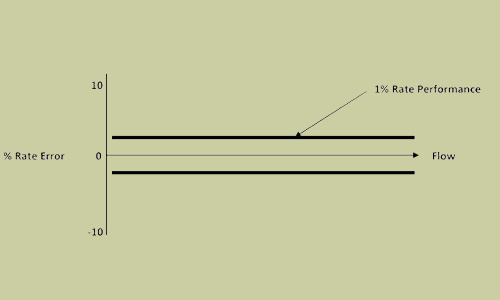As shown in the graph, the % Rate Error between the range of low flows and high flows stays constant.

### Percent of Full Scale (%FS):

The error in the calculation of flow rate while the accuracy of the meter is expressed as a percent of full scale may be determined by:

Error = % FS*Full Scale Flow

Assume a flow meter with a stated accuracy of ±1%FS. If the meter is being utilized to estimate liquid volume in the range 0 to100 gpm then we can determine the errors at 100%, 50%, 25% and 10% of the flow range as:

• At 100% of flow, error = ±0.01*100 = ±0.1 gpm = 1% rate
• 50% of flow, error = ±0.01*100 = ±0.1 gpm = 2% rate
• 25% of flow, error = ±0.01*100 = ±0.1 gpm = 4% rate
• 10% of flow, error = ±0.01*100 = ±0.1 gpm = 10% rate

Note that % R= error/measurement

As indicated in this example, when the error is constant at any given flow, the error stated as a percent of flow rate enhances with a reduction in flow rate. This isn't good because at low flow, the errors become high.

A plot of % R error against flow gives the graph below: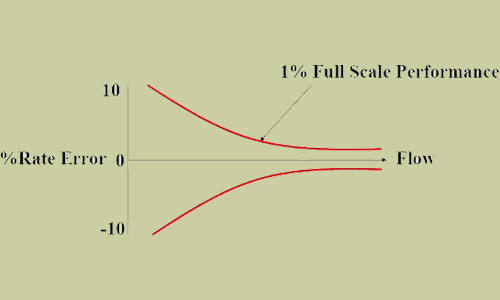### Percent of Calibrated Span (%CS):

The error in the measurement of flow rate while the accuracy of the meter is expressed as a percent of calibrated span may be determined by:

Error = % CS* Calibrated span

Assume a flow meter with an expressed accuracy of ±1%CS. If the meter is being utilized to measure liquid volume in the range 0 to100 gpm but with a calibrated span of 200 gpm and an upper range limit (URL) of 400 gpm then the errors may be determined at 100%, 50%, 25% and 10% of the flow range as:

• At 100% of flow, error = ±0.01*200 = ±2 gpm = 2% rate
• 50% of flow, error = ±0.01*200 = ±2 gpm = 4% rate
• 25% of flow, error = ±0.01*200 = ±2 gpm = 8% rate
• 10% of flow, error = ±0.01*200 = ±2 gpm = 20% rate

A plot of % R error against flow gives the graph below: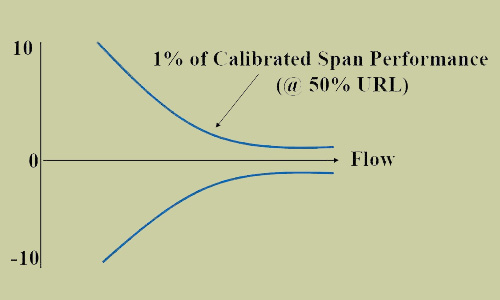### Percent of Upper Range Limit (%URL):

The error in the calculation of flow rate while the accuracy of the meter is expressed as a percent of upper range limit may be determined by:

Error = %URL*Upper range limit

Assume a flow meter with a stated accuracy of ±1%URL. If the meter is being utilized to estimate liquid volume in the range 0 to100 gpm but with an upper range limit (URL) OF 400 gpm then the errors can be determined at 100%, 50%, 25% and 10% of the flow range as:

• At 100% of flow, error = ±0.01*400 = ±4 gpm = 4% rate
• 50% of flow, error = ±0.01*400 = ±4 gpm = 8% rate
• 25% of flow, error = ±0.01*400 = ±4 gpm = 16% rate
• 10% of flow, error = ±0.01*400 = ±4 gpm = 40% rate

A plot of % R error against flow gives the graph below: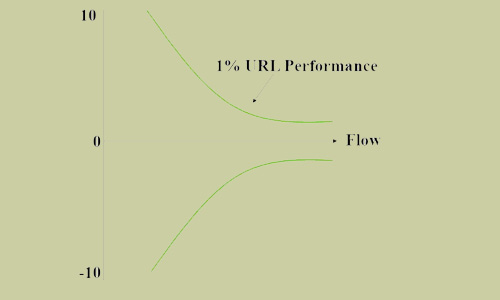A mixture of these graphs gives the graph below: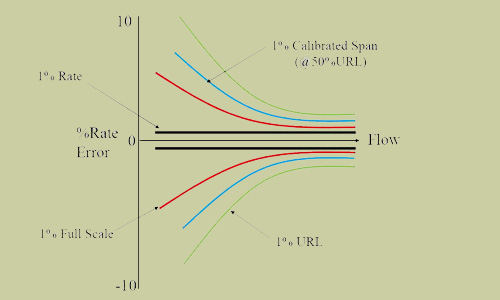A precise look at this single graph depicting all the different flow meter accuracy statements indicates that:

a) Flow meter accuracy stated as a percent of calibrated span (%CS) is just like full scale (%FS) and %URL accuracy statements where the absolute error is a percentage of the calibrated span.

b) a calibrated span statement will become a full scale statement while the instrument is calibrated to full scale.

C) a calibrated span statement will become a %URL statement when the device is calibrated at URL.

From the discussions so far, it ought to be mentioned that flow meter performance specific as a percent of rate (%R), percent of full scale (%FS), percent of URL (%URL), and percent of calibrated span (%CS) are unique and should not be confused with each other.

In preparing flow meter accuracy specifications therefore, all accuracy statements ought to be changed into uniform % actual flow rate and these % actual flow necessities ought to be specified one after the other for minimum, normal, and maximum flows.

So, we will truly appreciate the magnitude of the errors at the various flow levels-maximum, normal and maximum flows and hence determine which flow meter is the most appropriate for their application.

11th Jun 2022 Saeed Abd

• ## The Mighty Load Cells: Unlocking the Hidden Power of Force Measurement

Load cells, the unsung heroes of the measurement world, have revolutionized the way we quantify f …
27th Sep 2023
• ## The Ultimate Showdown: Load Cell Comparison Demystified

Welcome to the Load Cell Comparison Guide! If you're looking for a clear and concise way to under …
25th Sep 2023
• ## Revolutionizing Human-Machine Interaction: Unveiling the Power of HMI

Introduction In today's interconnected world, the seamless interaction between humans and machines …
20th Sep 2023